Next: Sub-Critical and Super-Critical Flow Up: Incompressible Inviscid Flow Previous: d'Alembert's Paradox

# Flow Through an Orifice

Consider the situation, illustrated in Figure 4.3, in which a horizontal jet of fluid emerges from an orifice in the side of a container. As shown in the figure, the jet narrows over a short distance beyond the orifice that is comparable with the jet diameter to form what is generally known as a vena contracta--that is, a contracted vein.'' The jet is bound to narrow in this manner because of the curvature of the lines of flow as they pass through the orifice. The narrowing of the jet implies the existence of a transverse pressure gradient. In other words, the pressure at, on the axis of the jet, is higher than the atmospheric pressure that acts at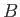. The pressure excess atsuggests that the fluid on the axis is still accelerating longitudinally as it leaves the orifice. Only in the vena contracta does the flow velocity becomes uniform, and the pressure atmospheric, all the way across the jet.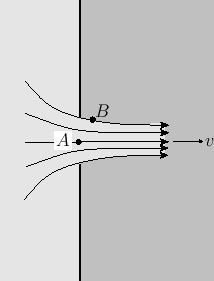Letbe the cross-sectional area of the orifice, and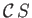that of the vena contracta. Here,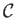is known as the contraction coefficient. Let us apply Bernoulli's theorem to a streamline that starts on the surface of the fluid within the container, and ends in the vena contracta. Suppose that the surface of the fluid lies a heightabove the orifice. Let us assume that the fluid close to the surface is essentially at rest (which implies that the outflow through the orifice is not sufficiently strong to cause the surface level to drop at a significant rate.) Let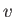be the uniform fluid velocity in the vena contracta. Of course, the pressure is atmospheric both at the surface of the fluid and in the vena contracta. It follows that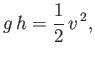(4.14)

or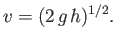(4.15)

In other words, the efflux velocity of the fluid from the orifice is the same as that it would have acquired by falling a heightunder gravity. This result is known as Torricelli's law, after Evangelista Torricelli (1608-1647). Finally, the discharge rate of fluid flowing through the orifice is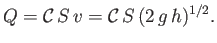(4.16)

Let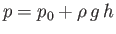be the hydrostatic pressure at the level of the orifice when the orifice is closed. Here,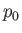is atmospheric pressure. The fluid experiences a thrust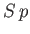from the section of the wall directly opposite the orifice, and a thrust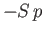from the section of the wall closing the orifice. Let us suppose, as a first approximation, that the hydrostatic pressure remains unaltered when the orifice is opened. In this situation, the fluid experiences a thrustfrom the section of the wall directly opposite the orifice, and a thrust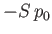from the orifice. The net thrust,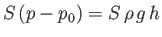, is responsible for accelerating the jet. Now, the jet's rate of momentum outflow is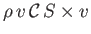. Momentum conservation yields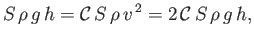(4.17)

where use has been made of Equation (4.15). Thus, we conclude that the contraction coefficient takes the value.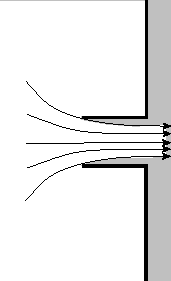In reality, Bernoulli's theorem suggests that when the orifice is opened the pressure on the walls in the neighborhood of the orifice will fall below the hydrostatic value, which implies that the accelerating thrust is actually greater than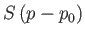. Consequently,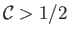. Obviously,cannot exceed unity, so we conclude that, in general,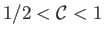. For instance, if the orifice is a circular hole punched in a thin plate then the contraction coefficient is observed to take the value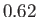(Batchelor 2000).

Suppose, however, that we fit a small cylindrical nozzle projecting inward from the orifice, as shown in Figure 4.4. In this case, the original assumption that the pressure on the walls in the neighborhood of the orifice is hydrostatic is essentially correct. This follows because the region where the lines of flow are converging on the orifice is far removed from the walls, and the velocity of the fluid in contact with the walls is negligible. Thus, the contraction coefficient is exactly. This arrangement is known as a Borda mouthpiece, after Jean-Charles Borda (1733-1799).Next: Sub-Critical and Super-Critical Flow Up: Incompressible Inviscid Flow Previous: d'Alembert's Paradox
Richard Fitzpatrick 2016-03-31